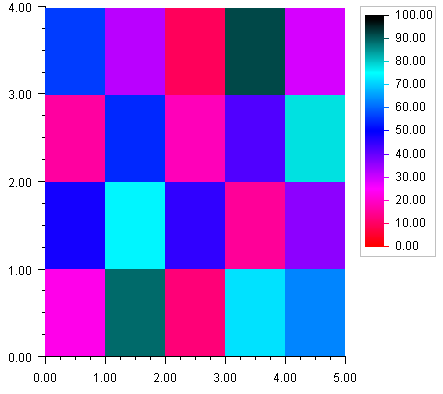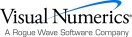## Heatmap

A Heatmap divides a rectangle into subrectangles. The color of each subrectangle is determined by the value of a data array and a colormap.

If the data array is m by n then there are m divisions along the x-axis and n divisions along the y-axis.

A Colormap is a mapping from [0,1] to color values. The blue-red colormap, used in the example below, maps 0 to red and 1 to dark blue and interpolates between these endpoints values. The heatmap maps the minimum data value to the color corresponding to 0 and the highest data value to the color corresponding to 1.

The Heatmap class has a special legend for colormaps. It displays the color values as a gradient labeled with corresponding data values.

### Example

In this example a two-dimensional array of data is plotted as a heatmap. The “red-blue” Colormap is used. The HeatmapLegend is enabled by setting its IsVisible property to true.using Imsl.Chart2D;

public class SampleHeatmap : FrameChart {

public SampleHeatmap() {

Chart chart = this.Chart;

AxisXY axis = new AxisXY(chart);

double xmin = 0.0;

double xmax = 5.0;

double ymin = 0.0;

double ymax = 4.0;

double zmin = 0.0;

double zmax = 100.0;

double[,] data = new double [,] {

{23, 48, 16, 56},

{89, 74, 54, 32},

{12, 45, 18,  9},

{72, 15, 42, 92},

{63, 36, 78, 29}

};

Heatmap heatmap = new Heatmap(axis, xmin, xmax, ymin, ymax,

zmin, zmax, data, Colormap_Fields.BLUE_RED);

heatmap.HeatmapLegend.IsVisible = true;

}

public static void Main(string[] argv) {

System.Windows.Forms.Application.Run(new SampleHeatmap());

}

}Visual Numerics - Developers of IMSL and PV-WAVEhttp://www.vni.com/PHONE: 713.784.3131FAX:713.781.9260